SCIA Engineer: Advanced use of Table input

Did you ever want to...

... rename several members at once ?

... round coordinates to a certain number of digits ?

... modify coordinates with simple mathematical formulas ?

... select objects in a table based on certain properties ?

... quickly show or hide specifics layers in an overview table ?

All this - and more - can be achieved with the powerful "Table input" functionality of SCIA Engineer !

Renaming several members at once

The Table input allows users to rename a member. When more than one name is selected in the table grid they are all renamed and also automatically renumbered (the name stays unique).

Example:
A set of names is selected in the Table input (on the 1D member tab). The new name of the first entity is typed in the Multiple editbox. In our example we used name “bbb_12”. All the selected entities are renamed. The first one is assigned name “bbb_12”, the second one “bbb_13”, the third one “bbb_14”, etc.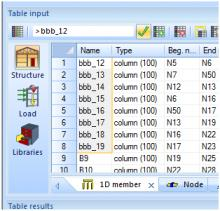Rounding values using Table input

After a structure has been imported to SCIA Engineer (from a third party program), it may sometimes contain little inaccuracies in coordinates. To be exact, the coordinate values contain too many digits after the decimal point - see the picture below.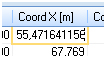This may cause problems or errors in subsequent work with the model.
Table input makes it possible to copy the content of the grid to the clipboard. Thus the user can easily copy the data to MS Excel (or another preferred spreadsheet) and round values there. The rounded data are then copied back to the Table input the same way – through the clipboard.
See the example in pictures:

1. The imported structure contains inaccurate coordinates in the X and Y direction.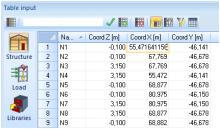2. The content is copied to the clipboard and pasted to MS Excel.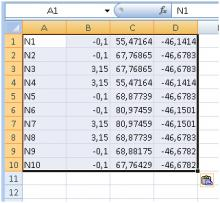3. The rounding
The function for rounding numbers (ROUNDUP / ROUNDDOWN in MS Excel) then allows you to specify the required number of decimal digits.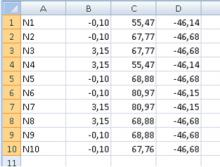4. The modified values are copied back to the Table input again through the clipboard. Now the grid contains rounded numbers and the model of the structure is better defined.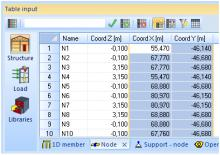Simple mathematical formulas in Table input

Numerical data in the Table input can also be modified using simple mathematical formulas. Numbers may be multiplied, divided, summed and subtracted. The editbox supports a simple syntax e.g. +3 means to add 3 to the selected value.
This functionality might be used e.g. to increase or decrease the load value on the structure in one step.

1. We need to increase all loads in Load case "LC6-columns" by 50 kN.
2. To do so the cells with the F values must be selected in the grid.
3.  Write "-50" to the multiple-editbox (the load value is negative, so we need to input a negative value to increase the absolute load size).
4. The action is confirmed by the Apply button - the new values are immediately calculated and all forces are increased (-650 kN -> -700 kN).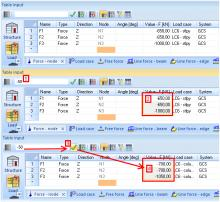Selection (highlighting) members according to a certain property

All members with the same property (e.g. the same cross-section) can be selected using a single click. This functionality is similar to filtering the model by a property in the Property window (the funnel icon).
How to select members with the same cross-section:

1.  Open the tab with 1D members.
2. Click on the cell with required cross-section.
3. Click on the button "Select by property in cell" on the toolbar.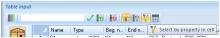4. All the members with the same cross-section are selected in the model (in the graphical window) and highlighted also in the Table input.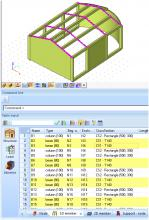Activity by layers in Table input (tab Layers)

The tab with Layers is very useful when a user wants to apply activity by layers in the model. The user can change the activity right here. The layer settings are visible all the time and the user is not forced to open the Layer manager when he needs to change the active layers.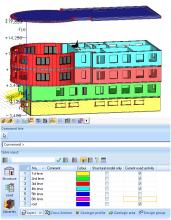Read more about Table input in the web-help for SCIA Engineer 14 (Basics / Layout and operation / User interface / Table input).